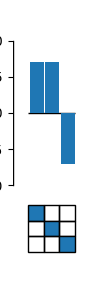Use a linear, logistic regression model to estimate the positive/negative effects of mutations.```# Imports
import matplotlib.pyplot as plt

from gpmap import GenotypePhenotypeMap
from epistasis.models import EpistasisLogisticRegression
from epistasis.pyplot import plot_coefs

# The data
wildtype = "000"
genotypes = ['000', '001', '010', '011', '100', '101', '110', '111']
phenotypes = [ 0.366, -0.593,  1.595, -0.753,  0.38 ,  1.296,  1.025, -0.519]
gpm = GenotypePhenotypeMap(wildtype, genotypes, phenotypes)

# Threshold
threshold = 1.0

# Initialize a model
model = EpistasisLogisticRegression(threshold=threshold)